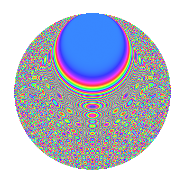# Properties

 Label 3024.2.erLevel 3024 Weight 2 Character orbit er Rep. character $$\chi_{3024}(253,\cdot)$$ Character field $$\Q(\zeta_{12})$$ Dimension 576 Sturm bound 1152

# Related objects

## Defining parameters

 Level: $$N$$ = $$3024 = 2^{4} \cdot 3^{3} \cdot 7$$ Weight: $$k$$ = $$2$$ Character orbit: $$[\chi]$$ = 3024.er (of order $$12$$ and degree $$4$$) Character conductor: $$\operatorname{cond}(\chi)$$ = $$144$$ Character field: $$\Q(\zeta_{12})$$ Sturm bound: $$1152$$

## Dimensions

The following table gives the dimensions of various subspaces of $$M_{2}(3024, [\chi])$$.

Total New Old
Modular forms 2352 576 1776
Cusp forms 2256 576 1680
Eisenstein series 96 0 96

## Trace form

 $$576q + O(q^{10})$$ $$576q + 28q^{20} - 64q^{26} + 56q^{38} - 48q^{46} + 288q^{49} - 52q^{50} + 36q^{58} + 24q^{59} + 24q^{62} + 72q^{64} + 52q^{68} + 28q^{74} - 24q^{76} + 304q^{80} + 72q^{82} + 124q^{86} + 48q^{88} - 76q^{92} + 128q^{95} + O(q^{100})$$

## Decomposition of $$S_{2}^{\mathrm{new}}(3024, [\chi])$$ into newform subspaces

The newforms in this space have not yet been added to the LMFDB.

## Decomposition of $$S_{2}^{\mathrm{old}}(3024, [\chi])$$ into lower level spaces

$$S_{2}^{\mathrm{old}}(3024, [\chi]) \cong$$ $$S_{2}^{\mathrm{new}}(144, [\chi])$$$$^{\oplus 4}$$$$\oplus$$$$S_{2}^{\mathrm{new}}(432, [\chi])$$$$^{\oplus 2}$$$$\oplus$$$$S_{2}^{\mathrm{new}}(1008, [\chi])$$$$^{\oplus 2}$$

## Hecke Characteristic Polynomials

There are no characteristic polynomials of Hecke operators in the database